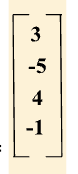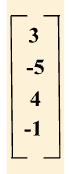# Row Matrix: Definition, Formula, Properties, Facts, Examples

## What Is a Row Matrix?

A row matrix is a type of a matrix that has only one row.

The total number of columns in a row matrix is the total number of elements that make up the single row

The row matrix is not a square matrix as the number of rows is not equal to the number of columns. Thus, we cannot find the determinant of a row matrix.

Examples of a row matrix:

A1 x 3 $= \left[7 \;5 \;6\right]$

B1 x 4 $= \left[10 \; 22 \; 63 \; 12\right]$

## Definition of Row Matrix

A row matrix is a matrix of an order $1 \times n$, where n is the number of columns.

A row matrix can be expressed in mathematical form as:

A = [a₁₁  a₁₂  a₁₃ ……. a₁ₙ]1 x n

where,

a₁₁ , a₁₂ , a₁₃ ,……., a₁ₙ are the elements

$n =$ number of columns

## Order of a Row Matrix

The order of a row matrix is $1 \times n$, where 1 represents the number of rows and n is the number of columns.

## Transpose of a Row Matrix

The transpose of a row matrix of order $1 \times n$ is a column matrix of order $n \times 1$. It is obtained by interchanging the row by a column. Transpose of a matrix A is denoted by A’ or AT.

Example: The transpose of the row matrix $A = \left[ 7 \;6 \;-\;9\right]$ is

## Properties of Row Matrix

1. There is only one row in a row matrix.
2. The total number of columns in a matrix equals the total number of elements in the row.
3. A row matrix is a type of a rectangular matrix.
4. A transpose of a row matrix with order $1 \times n$ is a column matrix with order $n \times 1$.
5. The row matrix can only be added to (or subtracted from) another row matrix with the same order.
6. A row matrix with order $1 \times n$ can only be multiplied by a column matrix of order $n \times 1$.
7. A singleton matrix (matrix of order $1 \times 1$ which has only one element) is produced when a row matrix and a column matrix are multiplied.

## Row Matrix Operations

We can perform simple arithmetic operations across a row matrix, such as addition, subtraction, multiplication, and division.

We can only add or subtract row matrices of the same order. To add/subtract two or more matrices, we add/subtract the corresponding elements.

Example:

$A = \left[3 9 8\right]$ and $B = \left[5 \; 1 \; 7\right]$

$A + B = \left[3 + 5 \; 9 + 1 \; 8 + 7\right] = \left[8 \; 10 \;15\right]$

$A \;-\; B = \left[3 \;-\; 5 \; 9 \;-\; 1 \; 8 \;-\; 7\right] = \left[\;-\;2 \; 8 \; 1\right]$

We can multiply a row matrix with a column matrix. We can multiply a row matrix of order $1 \times n$ only with a column matrix of order $n \times 1$. The result of the multiplication of a row and a column matrix is a singleton matrix.

Example:

Let us consider matrix $A = \left[2 \; 5 \;3\right]$ and

matrix B =

$A \times B = \left[(2 \times 7) + (5 \times 6) + (3\times -9)\right]$

$A \times B = \left[14 + 30 \;-\; 27\right]$

$A \times B = \left[44 \;-\; 27\right]$

$A \times B = \left[17\right]$

## Types of Matrices

There are ten different kinds of matrices. They are as follows:

1. Row matrix: A matrix that has only one row.
2. Column matrix: A matrix which contains only one column.
3. Diagonal matrix: A square matrix that has 0 as the element on the leading diagonals.
4. Scalar matrix: This kind of matrix has the same elements on the diagonal.
5. Square matrix: This type of matrix has the same number of rows and columns.
6. Identity matrix: It is also known as a unit matrix. A matrix with all the diagonal elements equal to 1.
7. Zero matrix: All the elements of this matrix are zero. It is also called a null matrix.
8. Triangular matrix: In this matrix, all the elements above or below the matrix leading diagonal are zero. There are two types of triangular matrix: upper triangular matrix and lower triangular matrix.
9. Symmetric matrix: A square matrix [aᵢⱼ] is a symmetric matrix when $a_{ij} = a_{ji}$.
10. Skew symmetric matrix: A skew-symmetric matrix is the kind of square matrix [aᵢⱼ] when $a_{ij} = \;-\;a_{ji}$. The elements of the leading diagonal of this matrix are 0.

• The division operation is not possible for a row matrix because the inverse of this type of matrix does not exist.
• A row matrix is also called a row vector in linear algebra.

## Conclusion

In this article, we learned about row matrices, their properties and also discussed operations on row matrices. Let’s use the concepts we learned to solve a few examples and practice problems.

## Solved Examples on Row Matrix

1. Find the sum of the row matrices $A = \left[4 \; 10 \; 11\right]$ and B $= \left[6\; 5 \; 20\right]$.

Solution: The given matrices are

$A = \left[4 \;10\; 11\right]$

$B = \left[6 \; 5 \; 20\right]$

$A + B = \left[4\; 10 \;11] + [6 \;5 \;20\right]$

$A + B = \left[4 + 6 \; 10 + 5 \; 11 + 20\right]$

$A + B = \left[10\; 15 \;31\right]$

Therefore, the resultant matrix $A + B = \left[10 \; 15\; 31\right]$.

2. Subtract matrix $B = \left[31 \; 25\; 16\right]$ from matrix $A = \left[47 \; 25 \; 34\right]$

Solution: The given matrices are:

$B = \left[31 \; 25 \; 16\right]$

$A = \left[47 \; 25 \; 34\right]$

$A \;-\; B = \left[47 25 \; 34\right] \;-\; \left[31 \;25 \; 16\right]$

$A \;-\; B = \left[47\;-\;31 \; 25\;-\;25 \; 31\;-\;16\right]$

$A \;-; B = \left[16\; 0 \; 15\right]$

Therefore, the resultant matrix would be $A \;-\; B = \left[16\; 0 \;15\right]$

3. Find the product of the following matrices $A = \left[2 \; 6 \;7 \;-\;5\right]$ and B =Solution: The given matrices are

$A = \left[2 \; 6 \;7 \;-\;5\right]$

B =$A \times B = \left[(2 \times 3) + (\;-\;5 \times 6) + (7 \times 4) + (\;-\;5 \times \;-\;1)\right]$

$A \times B = \left[6 \;-\; 30 + 28 + 5\right]$

$A \times B = \left[39 \;-\; 30\right]$

$A \times B = \left[9\right]$

Therefore, the product of the matrices is $A \times B = \left[9\right]$.

## Practice Problems on Row Matrix

1

### $M = \left[15 \;24 \; 30 \; 41\right]$ and $N = \left[20 \; 37 \; 28 \; 11\right]$. Find $M + N$.

$\left[30 \; 62 \; 58 \; 52\right]$
$\left[35 \; 61 \; 58\; 52\right]$
$\left[35 \; 61 \; 59 \;55\right]$
$\left[36 \; 61 \;58 \; 52\right]$
CorrectIncorrect
Correct answer is: $\left[35 \; 61 \; 58\; 52\right]$
When we add matrix M and N, we get
$M + N = \left[15 + 20 \; 24 + 37 \; 30 + 28 \; 41 + 11\right] = \left[35 \; 61 \; 58 \;52\right]$.
2

### The order of the row matrix $C = \left[6 \; 2 \; 1 \;3\right]$ is

$4 \times 4$
$6 \times 1$
$4 \times 1$
$1 \times 4$
CorrectIncorrect
Correct answer is: $1 \times 4$
The matrix has a single row and 4 columns. Thus, the order of the matrix $C = \left[6 \; 2 \; 1 \;3\right]$ is $1 \times 4$.
3

### Addition of zero matrix of order $1 \times 3$ and the matrix $\left[1 \; 2 \; 3\right]$ is

$\left[3 \; 2 \; 1\right]$
6
$\left[1 \; 2 \; 3\right]$
$\left[\;-\;1 \;-\;2 \;-\;3\right]$
CorrectIncorrect
Correct answer is: $\left[1 \; 2 \; 3\right]$
$\left[1 \; 2 \; 3\right] + \left[0 \;0 \;0\right] = \left[1 \;2 \;3\right]$